Home
 Links to Websites for Teaching Fractions . http://standards.nctm.org/document/eexamples/chap5/5.1/index.htm Mathematical games can foster mathematical communication as students explain and justify their moves to one another. In addition, games can motivate students and engage them in thinking about and applying concepts and skills. This first part, Playing Fraction Track, contains an interactive version of a game (based on the work of Akers, Tierney, Evans, and Murray ) that can be used in the grades 3–5 classroom to suport students' learning about fractions.By working on this activity, students have opportunities to think about how fractions are related to a unit whole, compare fractional parts of a whole, and find equivalent fractions, as discussed in the Number and Operations Standard. In the second part, The Role of the Teacher, two video clips illustrate communication about mathematics among a teacher and her students. The third part, Communication among Students, shows how activities like this allow students to use communication as a tool to deepen their understanding of mathematics, as described in the Communication Standard. In the fourth part, Reflecting on Practice, the teacher reflects on her own mathematical learning that occurs as a result of using activities like this game with her fifth-grade students. http://standards.nctm.org/document/eexamples/chap6/6.1/index.htm Contains examples for grades: Pre-K-2, 3-5, 6-8, and 9-12. The example shown here pertains to multiplying fractions and decimals. Learning about Multipliction Students can learn to visualize the effects of multiplying a fixed positive number by positive numbers greater than 1 and less than 1 with this tool. Using interactive figures, students can investigate how changing the height of a rectangle with a fixed width changes its area.As discussed in the Number Standard, understanding multiplication by fractions and decimals can be challenging for middle-grades students if experiences with multiplication by whole numbers have led them to believe that "multiplication makes bigger." In these dynamic figures, the rectangle represents the familiar area model of multiplication; changing the rectangle's height can help students see the effect of multiplying a fixed positive number by numbers greater than 1 and less than 1. http://www.fractionbars.com Contains NCTM articles on fractions, links to websites for teaching fractions, examples from National Assessment of Educational Progress and NCTM's Standards, eight interactive games for learning about fractions, and details and examples from the Fraction Bars Program. .http://www.educationworld.com/a_lesson/03/lp303-05.shtml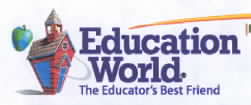Students read simple music to create a symphony of clapping (all grades). Plus fraction math for grades 5-up. Work sheet included. http://illuminations.nctm.org/ActivityDetail.aspx?ID=18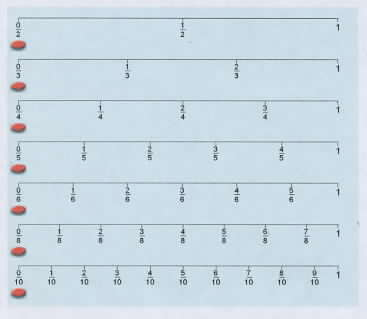This applet allows students to individually practice working with relationships among fractions and ways of combining fractions. . http://www.geocities.com/smilecdg/fractionles.html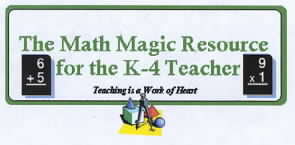This link has many links to teacher-made lessons on teaching fractions. http://www.gamequarium.com/fractions.html This link has many files and touches on the topics of equality, common denominators, lowest terms, and the four basic operations on fractions.http://www.lessonplanspage.com/This URL produces a home page and clicking on Search near the top of the screen and typing the word Fraction will produce many lesson plans for teaching fractions. http://math.rice.edu/~lanius/Lessons/Series/infinite.htmVisualizing an infinite sum of fractions with pattern blocks. http://standards.nctm.org/document/chapter3/index.htmThis is the standards chapter in the Principles and Standards for School Mathematics of the National Council of Teachers of Mathematics. Select Number and Operations from the left column for across-the-grades standards. Information on Fractions can be found at grade levels 3 – 5 and 6 – 8, from the top row. http://www.decimalsquares.com Contains NCTM articles on decimals, links to websites for teaching decimals, examples from National Assessment of Educational Progress and NCTM's Standards, eight interactive games for learning about decimals, and details and examples from the Decimal Squares Program. In particular, the file Relating Decimals to Fractions ( /relatingdecsfracs.html) has activities for demonstrating relationships between the learning of decimals and fractions.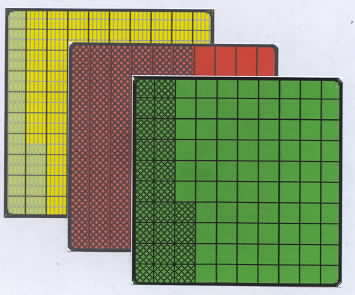http://www.aaamath.com The files on this site have operations on fractions from K-8 math courses. There are explanations, interactive practice and games involving fractions.Home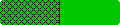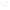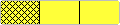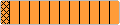Home